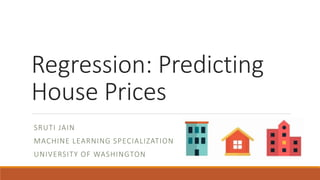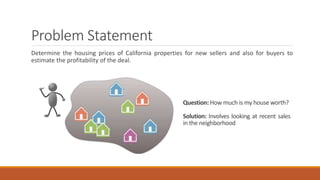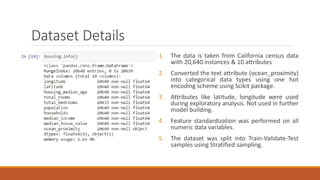Successfully reported this slideshow.

# Predicting house prices_Regression×

1 of 12 Ad
1 of 12 Ad

# Predicting house prices_Regression

This project aims to determine the housing prices of California properties for new sellers and also for buyers to estimate the profitability of the deal using various regression models.
Below are the details of the models implemented and their performance score:
Linear Regression: RMSE- 68321.7051304
Decision Tree Regressor: RMSE- 70269.5738668
Random Forest Regressor: RMSE- 52909.1080535
Support Vector Regressor: RMSE- 110914.791356
Fine Tuning the Hyperparameters for Random Forest Regressor: RMSE- 49261.2835608

This project aims to determine the housing prices of California properties for new sellers and also for buyers to estimate the profitability of the deal using various regression models.
Below are the details of the models implemented and their performance score:
Linear Regression: RMSE- 68321.7051304
Decision Tree Regressor: RMSE- 70269.5738668
Random Forest Regressor: RMSE- 52909.1080535
Support Vector Regressor: RMSE- 110914.791356
Fine Tuning the Hyperparameters for Random Forest Regressor: RMSE- 49261.2835608

### Predicting house prices_Regression

1. 1. Regression: Predicting House Prices SRUTI JAIN MACHINE LEARNING SPECIALIZATION UNIVERSITY OF WASHINGTON
2. 2. Problem Statement Determine the housing prices of California properties for new sellers and also for buyers to estimate the profitability of the deal. Question: How much is my house worth? Solution: Involves looking at recent sales in the neighborhood
3. 3. Dataset Details 1. The data is taken from California census data with 20,640 instances & 10 attributes 2. Converted the text attribute (ocean_proximity) into categorical data types using one hot encoding scheme using Scikit package. 3. Attributes like latitude, longitude were used during exploratory analysis. Not used in further model building. 4. Feature standardization was performed on all numeric data variables. 5. The dataset was split into Train-Validate-Test samples using Stratified sampling.
4. 4. Correlation Plot
5. 5. Exploratory Analysis Plot Plot to visualize role of latitude, longitude & population on the price of the house
6. 6. Training-Testing Models 1. Linear Regression 2. Decision Tree Regressor 3. Random Forest Regressor 4. Support Vector Regressor 5. Fine Tuning the Hyperparameters for Random Forest Regressor using Grid Search and Randomized Search Note: Random seed values were picked to develop training, validation & testing sets in the ratio 60:20:20
7. 7. Linear Regression Linear regression helped understand which variable are significant & which not. Also since many of our attributes are continuous, linear regression is a good approach to use as a starting step.
8. 8. Decision Tree Regressor
9. 9. Random Forest Regressor
10. 10. Support Vector Regressor
11. 11. Comparative Analysis 1. In multiple linear regression, the best R-Squared 0.6002, correlation of prediction and test is 0.7748672 and RMSE- 68321.70. 2. In Decision Tree, the best regression model comes from random forest with correlation 0.876914 and RMSE- 70269.57. 3. In SVM model, model with linear kernel performs best with correlation 0.82014 & RMSE- 110914.79. 4. Of the four models, random forest performs better than the others with least RMSE- 49261.28 obtained by tuning the Hyperparameters using Randomized Search.
12. 12. Thank You !!